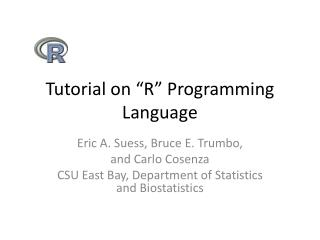DownloadDownload PresentationTutorial on “R” Programming Language

# Tutorial on “R” Programming Language

Télécharger la présentation## Tutorial on “R” Programming Language

- - - - - - - - - - - - - - - - - - - - - - - - - - - E N D - - - - - - - - - - - - - - - - - - - - - - - - - - -
##### Presentation Transcript

1. Tutorial on “R” Programming Language Eric A. Suess, Bruce E. Trumbo, and Carlo Cosenza CSU East Bay, Department of Statistics and Biostatistics

2. Outline • Communication with R • R software • R Interfaces • R code • Packages • Graphics • Parallel processing/distributed computing • Commerical R REvolutions

3. Communication with R • In my opinion, the R/S language has become the most common language for communication in the fields of Statistics and and Data Analysis. • Books are being written now with R presented directly placed within the text. • SV use R, for example • Excellent for teaching.

4. R Software • To download R • http://www.r-project.org/ • CRAN • Manuals • The R Journal • Books

5. R Software

6. R Interfaces • RWinEdt • Tinn-R • JGR (Java Gui for R) • Emacs + ESS • Rattle • AKward • Playwith (for graphics)

7. R code > 2+2  4 > 2+2^2  6 > (2+2)^2  16 > sqrt(2)  1.414214 > log(2)  0.6931472 > x = 5 > y = 10 > z <- x+y > z  15

8. R Code > seq(1,5, by=.5)  1.0 1.5 2.0 2.5 3.0 3.5 4.0 4.5 5.0 > v1 = c(6,5,4,3,2,1) > v1  6 5 4 3 2 1 > v2 = c(10,9,8,7,6,5) > > v3 = v1 + v2 > v3  16 14 12 10 8 6

9. R code > max(v3);min(v3)  16  6 > length(v3)  6 > mean(v3)  11 > sd(v3)  3.741657

10. R code > v4 = v3[v3>10] > v4  16 14 12 > n = 1:10000; a = (1 + 1/n)^n > cbind(n,a)[c(1:5,10^(1:4)),] n a [1,] 1 2.000000 [2,] 2 2.250000 [3,] 3 2.370370 [4,] 4 2.441406 [5,] 5 2.488320 [6,] 10 2.593742 [7,] 100 2.704814 [8,] 1000 2.716924 [9,] 10000 2.718146

11. R code # LLN cummean = function(x){ n = length(x) y = numeric(n) z = c(1:n) y = cumsum(x) y = y/z return(y) } n = 10000 z = rnorm(n) x = seq(1,n,1) y = cummean(z) X11() plot(x,y,type= 'l',main= 'Convergence Plot')

12. R code # CLT n = 30 # sample size k = 1000 # number of samples mu = 5; sigma = 2; SEM = sigma/sqrt(n) x = matrix(rnorm(n*k,mu,sigma),n,k) # This gives a matrix with the samples # down the columns. x.mean = apply(x,2,mean) x.down = mu - 4*SEM; x.up = mu + 4*SEM; y.up = 1.5 hist(x.mean,prob= T,xlim= c(x.down,x.up),ylim= c(0,y.up),main= 'Sampling distribution of the sample mean, Normal case') par(new= T) x = seq(x.down,x.up,0.01) y = dnorm(x,mu,SEM) plot(x,y,type= 'l',xlim= c(x.down,x.up),ylim= c(0,y.up))

13. R code # Birthday Problem m = 100000; n = 25 # iterations; people in room x = numeric(m) # vector for numbers of matches for (i in 1:m) { b = sample(1:365, n, repl=T) # n random birthdays in ith room x[i] = n - length(unique(b)) # no. of matches in ith room } mean(x == 0); mean(x) # approximates P{X=0}; E(X) cutp = (0:(max(x)+1)) - .5 # break points for histogram hist(x, breaks=cutp, prob=T) # relative freq. histogram

14. R help • help.start() Take a look • An Introduction to R • R Data Import/Export • Packages • data() • ls()

15. R code Data Manipulation with R (Use R) Phil Spector

16. R Packages • There are many contributed packages that can be used to extend R. • These libraries are created and maintained by the authors.

17. R Package - simpleboot mu = 25; sigma = 5; n = 30 x = rnorm(n, mu, sigma) library(simpleboot) reps = 10000 X11() median.boot = one.boot(x, median, R = reps) #print(median.boot) boot.ci(median.boot) hist(median.boot,main="median")

18. R Package – ggplot2 • The fundamental building block of a plot is based on aesthetics and facets • Aesthetics are graphical attributes that effect how the data are displayed. Color, Size, Shape • Facets are subdivisions of graphical data. • The graph is realized by adding layers, geoms, and statistics.

19. R Package – ggplot2 library(ggplot2) oldFaithfulPlot = ggplot(faithful, aes(eruptions,waiting)) oldFaithfulPlot + layer(geom="point") oldFaithfulPlot + layer(geom="point") + layer(geom="smooth")

20. R Package – ggplot2 Ggplot2: Elegant Graphics for Data Analysis (Use R) Hadley Wickham

21. R Package - BioC • BioConductor is an open source and open development software project for the analysis and comprehension of genomic data. • http://www.bioconductor.org • Download > Software > Installation Instructions source("http://bioconductor.org/biocLite.R") biocLite()

22. R Package - affyPara library(affyPara) library(affydata) data(Dilution) Dilution cl <- makeCluster(2, type='SOCK') bgcorrect.methods() affyBatchBGC <- bgCorrectPara(Dilution, method="rma", verbose=TRUE)

23. R Package - snow • Parallel processing has become more common within R • snow, multicore, foreach, etc.

24. R Package - snow • Birthday Problem simulation in parallel cl <- makeCluster(4, type='SOCK') birthday <- function(n) { ntests <- 1000 pop <- 1:365 anydup <- function(i) any(duplicated( sample(pop, n,replace=TRUE))) sum(sapply(seq(ntests), anydup)) / ntests} x <- foreach(j=1:100) %dopar% birthday (j) stopCluster(cl) Ref: http://www.rinfinance.com/RinFinance2009/presentations/UIC-Lewis%204-25-09.pdf

25. REvolution Computing • REvolution R is an enhanced distribution of R • Optimized, validated and supported • http://www.revolution-computing.com/